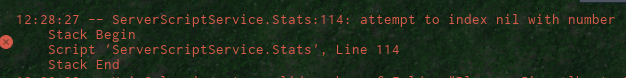I’ve been trying to load a characters HairColor value for a character customization. The problem is that the game has trouble loading their data, here is the code and the error i got.``````
-- Data Store Stuff

local DSS = game:GetService("DataStoreService"):GetDataStore("DemonSlayer")

function save(plr)
local s = {}
for i, v in pairs(plr:WaitForChild("Data"):GetChildren()) do
if v.Name == "HairColor" then
local rgb = {v.Value.R, v.Value.G, v.Value.B}
s[v.Name] = rgb
else
s[v.Name] = v.Value
end
end

DSS:SetAsync(plr.UserId, s)
end
--------------------------------------------------------

local ServerScriptService = game:GetService("ServerScriptService")

local plrData = DSS:GetAsync(player.UserId) -- Load saved data from the data store

local data = Instance.new("Folder")
data.Name = "Data"
data.Parent = player

local Strength = Instance.new("IntValue")
Strength.Name = "Strength"
Strength.Value = 4
Strength.Parent = data

local def = Instance.new("IntValue")
def.Name = "Defense"
def.Value = 0
def.Parent = data

local br = Instance.new("IntValue")
br.Name = "BreathLevel"
br.Value = plrData.BreathLevel or 1
br.Parent = data

local lastname = Instance.new("StringValue")    --Last Name
lastname.Name = "Bloodline"
lastname.Value = plrData.Bloodline or "None"
lastname.Parent = data

local Rank = Instance.new("StringValue")
Rank.Name = "Rank"
Rank.Value = plrData.Rank or "Civilian"
Rank.Parent = data

local Race = Instance.new("StringValue")
Race.Name = "Race"
Race.Value = plrData.Race or "Human"
Race.Parent = data

local FN = Instance.new("StringValue")   --First Name
FN.Name = "Name"
FN.Value = plrData.Name or "None"
FN.Parent = data

local spin = Instance.new("IntValue")
spin.Name = "Spins"
spin.Value = plrData.Spins or 5
spin.Parent = data

local hr = Instance.new("IntValue")
hr.Name = "Hair"
hr.Value = plrData.Hair or 1
hr.Parent = data

local hr2 = Instance.new("IntValue")
hr2.Name = "Hair2"
hr2.Value = plrData.Hair2 or 0
hr2.Parent = data

local sh = Instance.new("IntValue")
sh.Name = "Shirt"
sh.Value = plrData.Shirt or 0
sh.Parent = data

local pn = Instance.new("IntValue")
pn.Name = "Pants"
pn.Value = plrData.Pants or 0
pn.Parent = data

local ey = Instance.new("IntValue")
ey.Name = "Eye"
ey.Value = 0
ey.Parent = data

local speed = Instance.new("IntValue")
speed.Name = "Speed"
speed.Value = 16
speed.Parent = data

local GN = Instance.new("StringValue")
GN.Name = "Gender"
GN.Value = plrData.Gender or "None"
GN.Parent = data

local HairColor = Instance.new("Color3Value")
HairColor.Name = "HairColor"
HairColor.Value = Color3.new(plrData.HairColor, plrData.HairColor, plrData.HairColor) or Color3.new(0,0,0)    --line 114
HairColor.Parent = data

----------------------------------------------------------

--------------------------------------------------

local success, errorm = pcall(function()

for i2, v2 in pairs(v)do
if data:FindFirstChild(i2) then
data:FindFirstChild(i2).Value = v2
end
end
end

end

end)

game:BindToClose(function()
for i, v in pairs(game.Players:GetChildren())do
save(v)
end
end)

game.Players.PlayerRemoving:Connect(function(Player)
save(Player)

end)
end)

``````

What is line 114? When I plug it into studio it is telling me “HairColor.Parent = data” which shouldn’t be right…

``````HairColor.Value = Color3.new(plrData.HairColor, plrData.HairColor, plrData.HairColor) or Color3.new(0,0,0)
``````

You did not “pcall” your plrData. Do something like:

``````
local plrData

local success = pcall(function()

plrData = DSS:GetAsync(player.UserId)

end)

if not plrData then

--make default plrData here

end
``````

So would I do something like this or would it have to be different?

``````-- Data Store Stuff

local DSS = game:GetService("DataStoreService"):GetDataStore("DemonSlayer")

function save(plr)
local s = {}
for i, v in pairs(plr:WaitForChild("Data"):GetChildren()) do
if v.Name == "HairColor" then
local rgb = {v.Value.R, v.Value.G, v.Value.B}
s[v.Name] = rgb
else
s[v.Name] = v.Value
end
end

DSS:SetAsync(plr.UserId, s)
end
--------------------------------------------------------

local ServerScriptService = game:GetService("ServerScriptService")

local plrData = DSS:GetAsync(player.UserId) -- Load saved data from the data store

local success = pcall(function()

local data = Instance.new("Folder")
data.Name = "Data"
data.Parent = player

local Strength = Instance.new("IntValue")
Strength.Name = "Strength"
Strength.Value = 4
Strength.Parent = data

local def = Instance.new("IntValue")
def.Name = "Defense"
def.Value = 0
def.Parent = data

local br = Instance.new("IntValue")
br.Name = "BreathLevel"
br.Value = plrData.BreathLevel or 1
br.Parent = data

local lastname = Instance.new("StringValue")    --Last Name
lastname.Name = "Bloodline"
lastname.Value = plrData.Bloodline or "None"
lastname.Parent = data

local Rank = Instance.new("StringValue")
Rank.Name = "Rank"
Rank.Value = plrData.Rank or "Civilian"
Rank.Parent = data

local Race = Instance.new("StringValue")
Race.Name = "Race"
Race.Value = plrData.Race or "Human"
Race.Parent = data

local FN = Instance.new("StringValue")   --First Name
FN.Name = "Name"
FN.Value = plrData.Name or "None"
FN.Parent = data

local spin = Instance.new("IntValue")
spin.Name = "Spins"
spin.Value = plrData.Spins or 5
spin.Parent = data

local hr = Instance.new("IntValue")
hr.Name = "Hair"
hr.Value = plrData.Hair or 1
hr.Parent = data

local hr2 = Instance.new("IntValue")
hr2.Name = "Hair2"
hr2.Value = plrData.Hair2 or 0
hr2.Parent = data

local sh = Instance.new("IntValue")
sh.Name = "Shirt"
sh.Value = plrData.Shirt or 0
sh.Parent = data

local pn = Instance.new("IntValue")
pn.Name = "Pants"
pn.Value = plrData.Pants or 0
pn.Parent = data

local ey = Instance.new("IntValue")
ey.Name = "Eye"
ey.Value = 0
ey.Parent = data

local speed = Instance.new("IntValue")
speed.Name = "Speed"
speed.Value = 16
speed.Parent = data

local GN = Instance.new("StringValue")
GN.Name = "Gender"
GN.Value = plrData.Gender or "None"
GN.Parent = data

local HairColor = Instance.new("Color3Value")
HairColor.Name = "HairColor"
HairColor.Value = Color3.new(plrData.HairColor, plrData.HairColor, plrData.HairColor)
HairColor.Parent = data

if not plrData then

print("Making Data")
local HairColor = Instance.new("Color3Value")
HairColor.Name = "HairColor"
HairColor.Value = Color3.new(0,0,0)
HairColor.Parent = data
end

----------------------------------------------------------

--------------------------------------------------

local success, errorm = pcall(function()

for i2, v2 in pairs(v)do
if data:FindFirstChild(i2) then
data:FindFirstChild(i2).Value = v2
end
end
end

end

end)

game:BindToClose(function()
for i, v in pairs(game.Players:GetChildren())do
save(v)
end
end)

game.Players.PlayerRemoving:Connect(function(Player)
save(Player)

end)
end)
end)```````

Uh… no Do what my code did, just in the if notplrData then condition, set plrData to a default. Normally when I do datastore, I would set the data after but in this case its a bit different. You can check this post if you want to see how I normally do it (its pretty basic but it gets the job done): Datastore Tutorial for Beginners

1 Like

Ohhh, I misread it, thank you so much! this also helps organize everything ^^